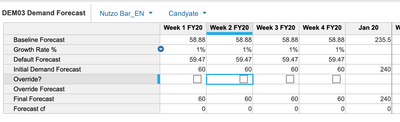# Level 2 Sprint 3 INV01 - Line Item FormulasHi,

I am stuck with the following formula that I don't seem to understand what it is looking for:

Forecast Demand line item

This line item should display the Final Forecast value from the DEM03 Demand Forecast module.

I have checked against my DEM03 formula (from Level 2) and all the formulas/values are right. My current answer is to just equate Final Forecast value to Forecast Demand but the number doesn't tally.

Any hints will be helpful. Thanks!

•Can you please share a screenshot of what doesn't look right to you. If I have to take a guess I would say you might be seeing aggregated values of SKUs for all the accounts in INV01 module because DEM03 has SKU and Account as dimensions wheres INV01 has only SKU as a dimension.

If not please post a snip here. Will try n help

Misbah

•Greetings,

In "DEM03 Demand Forecast" module select "All Accounts" in the Page Selector then compare "Final Forecast" of "DEM03 Demand Forecast" module with "Forecast Demand" of "INV01 Inventory Ordering" module. It should match.

Hope this helps.

•Hi @Misbah and @ANS ,

My DEM03-Final Forecast number tallies with my INV01-Forecast Demand (1,201) but doesn't look right with the reviewed example provided in the lesson (2,048) in Week 1 FY20 and beyond. I have attached screenshots of my work in the first 2 screenshots and the example given by the lesson in the third screenshot.

•Hey @JoanneN

The way I'd "debug" an issue like this is by examining and tracing back the formula for the mismatch value until I find a value/formula that may be the reason for the discrepancy.

By examining the formulas of the Line Item and trace it back, Here is how the math works for the INV01.Forecast Demand

• INV01. Forecast Demand = DEM03.Final Forecast
• DEM03.Final Forecast = Initial Demand OR Override (I assume you don't have Override so we'll use Initial Demand)
• DEM03.Initial Demand = Round(Base Line Demand)

The Value of INV01.Forecast Demand is really dependant on the value of DEM03.Base Line Demand.

Are you sure the formula for Base Line Demand is correct?
What value do you have for Nutzo Bar_EN for All Accounts in Week 1 FY20?

•Thanks for the guidance. I have checked my steps according to your advice and it seems like my data matches for DEM03 verification in Sprint 2.  My initial demand forecast for Week 1 FY20 is 60.

I am looking at  ROUND(Default Forecast, 0, UP) for initial demand. Am I missing something? Thanks!•Hi @JoanneN

Ok, is it likely that this formula is not working correctly? probably not, whatever value you give the formula it'll generate the roundup value. so you need to go one step further.

Default Forecast formula is dependant on Baseline Forecast

I'd look at the formula of Baseline Forecast and ensure it returns the correct value for the different time periods.

Note: Please note that the Forecast Demand in INV01 is for a particular P3 SKU in ALL accounts

•Hi Joanne,

Did you have the question figured out? I was looking for the solution too.

Also, my formula for DEM03 final forecast is IF Override? THEN Override Forecast ELSE Initial Demand Forecast

It appears to be correct in the module but when I use this in the exam it is wrong.

I really appreciate it if you can help with this question.